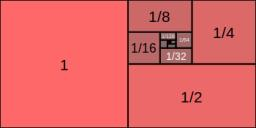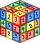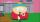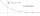# Gp - 80

Sum of the first four members of a geometric progression is 80. Determine they if we know that the fourth member is nine times greater than the second.

Correct result:

a1 =  2
a2 =  6
a3 =  18
a4 =  54
b1 =  -4
b2 =  12
b3 =  -36
b4 =  108

#### Solution:

${b}_{1}=80\mathrm{/}\left(1+{q}_{2}+{q}_{2}^{2}+{q}_{2}^{3}\right)=80\mathrm{/}\left(1+\left(-3\right)+\left(-3{\right)}^{2}+\left(-3{\right)}^{3}\right)=-4$We would be pleased if you find an error in the word problem, spelling mistakes, or inaccuracies and send it to us. Thank you!## Next similar math problems:

• Three members GPThe sum of three numbers in GP (geometric progression) is 21 and the sum of their squares is 189. Find the numbers.
• Geometric progressiobIf the sum of four consective terms of geometric progression is 80 and arithmetic mean of second and fourth term is 30 then find terms?
• GP - 8 itemsDetermine the first eight members of a geometric progression if a9=512, q=2
• GP - three membersThe second and third of a geometric progression are 24 and 12(c+1) respectively, given that the sum of the first three terms of progression is 76 determine value of c
• Fifth memberDetermine the fifth member of the arithmetic progression, if the sum of the second and fifth members equal to 73, and difference d = 7.
• Sum of membersWhat is the sum of the first two members of the aritmetic progression if d = -4.3 and a3 = 7.5?
• If the 3If the 6th term of a GP is 4 and the 10th is 4/81, find common ratio r.
• Geometric sequence 5About members of geometric sequence we know: ? ? Calculate a1 (first member) and q (common ratio or q-coefficient)
• QuotientDetermine the quotient and the second member of the geometric progression where a3=10, a1+a2=-1,6 a1-a2=2,4.
• Geometric sequence 3In geometric sequence is a8 = 312500; a11= 39062500; sn=1953124. Calculate the first item a1, quotient q and n - number of members by their sum s_n.
• QuizQ2The square of the first number is equal to three-fifths of the second number. Determine both numbers if you know that the second number is 5 times greater than the first, and neither of numbers is not equal to zero.
• Geometric progressionIn geometric progression, a1 = 7, q = 5. Find the condition for n to sum first n members is: sn≤217.
• Geometric sequenceIn the geometric sequence is a4 = 20 a9 = -160. Calculate the first member a1 and quotient q.
• Five membersWrite first 5 members geometric sequence and determine whether it is increasing or decreasing: a1 = 3 q = -2
• Sum of GP membersDetermine the sum of the GP 30, 6, 1.2, to 5 terms. What is the sum of all terms (to infinity)?
• Three numbersFind three numbers so that the second number is 4 times greater than the first and the third is lower by 5 than the second number. Their sum is 67.
• GP membersThe geometric sequence has 10 members. The last two members are 2 and -1. Which member is -1/16?